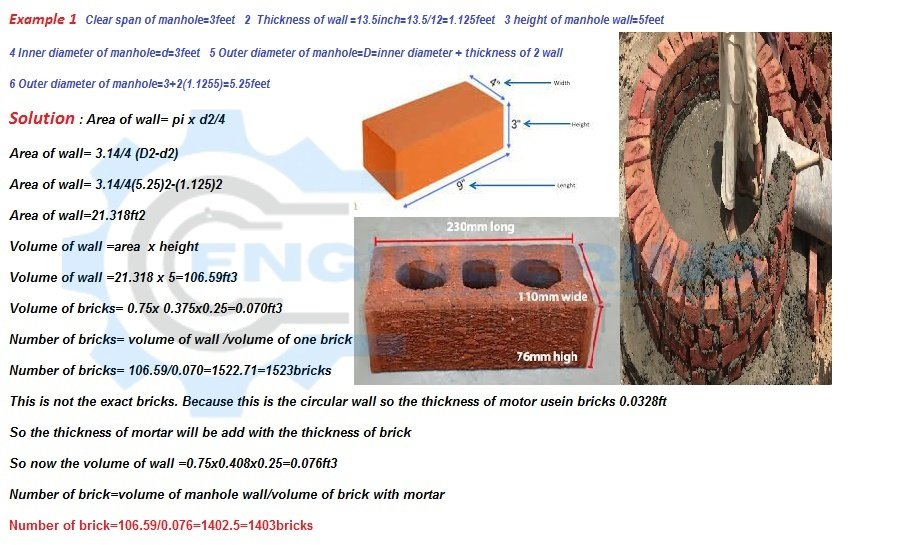Quantity Surveying

# Calculate The Quantity Of Bricks For Circular Manhole

Today In this article, I explain the manhole types of the manhole. Calculate The Quantity Of Bricks For the Circular Manhole.### Calculate The Quantity Of Bricks For Circular Manhole

The basic three types of manholes according to construction

Normal manhole

Shallow manhole

Deep manhole

### Normal Manhole

The depth of this manhole is up to 1.5meter or 5 feet. Normally the shape of this manhole is rectangle or square. This manhole is used for drainage water lines.

### Shallow Manhole

The depth of this manhole is up to 0.70meter to 0.90meter. this manhole is constructed is normally the starting point. This manhole is best for maintenance work.

### Deep Manhole

The depth of this manhole is greater than the 1.5m.this manhole has always heavy cover and also has this manhole the enter and exit point.

Now we calculate the number of bricks for circular manholeExample

Clearspan of manhole=3feet

Thickness of wall =13.5inch=13.5/12=1.125feet

Height of manhole wall=5feet

Inner diameter of manhole=d=3feet

Outer diameter of manhole=D=inner diameter + thickness of 2 wall

Outer diameter of manhole=3+2(1.1255)=5.25feet

### Solution

Area of wall= πd²/4

Area of wall= π(D²-d²)/4

Area of wall= 3.14(5.25)²-(1.125)²/4

Area of wall=21.318ft²

Volume of wall =area  x height

Volume of wall =21.318 x 5=106.59ft³

Volume of bricks= 0.75x 0.375×0.25=0.070ft³

Number of bricks= volume of wall /volume of one brick

Number of bricks= 106.59/0.070=1522.71=1523bricks

There are not the exact bricks. Because this is the circular wall so the thickness of the motor used in bricks is 0.0328ft

So the thickness of mortar will be added with the thickness of brick

So now the volume of wall =0.75×0.408×0.25=0.076ft³

Number of brick=volume of manhole wall/volume of a brick with mortar

Number of brick=106.59/0.076=1402.5=1403bricks